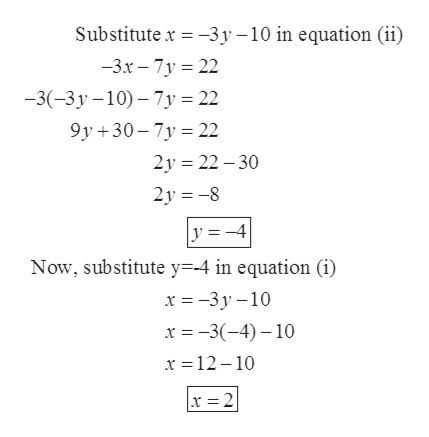# For Exercise, solve the system of equations usinga. The substitution method or the addition method b. Gaussian elimination c.Gauss-Jordan elimination d. The inverse of the coefficient matrix .e. Cramer’s rule .x =-3y-10-3x-7y=22

Question
6 views

For Exercise, solve the system of equations using

a. The substitution method or the addition method

b. Gaussian elimination

c.Gauss-Jordan elimination

d. The inverse of the coefficient matrix .

e. Cramer’s rule .

x =-3y-10

-3x-7y=22

check_circle

Step 1

Since we only answer up to 3 sub-parts, we’ll answer the first 3. Please resubmit the question and specify the other subparts (up to 3) you’d like answered.

Step 2

Given: solve the system of equations using

1. The substitution method or the addition method
2. Gaussian elimination
3. Gauss-Jordan elimination
Step 3

(a) using substitu...help_outlineImage TranscriptioncloseSubstitute x = -3y – 10 in equation (ii) -3.x – 7y = 22 -3(-3y – 10) – 7y = 22 9y +30– 7y = 22 2y = 22 – 30 2y = -8 y =-4 Now, substitute y=-4 in equation (i) x = -3y – 10 x = -3(-4) – 10 x = 12 – 10 x =2 fullscreen

### Want to see the full answer?

See Solution

#### Want to see this answer and more?

Solutions are written by subject experts who are available 24/7. Questions are typically answered within 1 hour.*

See Solution
*Response times may vary by subject and question.
Tagged in

### Equations and In-equations